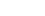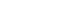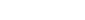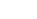How Cheenta works to ensure student success?
Explore the Back-Story

# TIFR 2015 Problem 4 Solution - Groups without Commuting ElementsTIFR 2015 Problem 4 Solution is a part of TIFR entrance preparation series. The Tata Institute of Fundamental Research is India's premier institution for advanced research in Mathematics. The Institute runs a graduate programme leading to the award of Ph.D., Integrated M.Sc.-Ph.D. as well as M.Sc. degree in certain subjects.
The image is a front cover of a book named Topics in Algebra by I.N.Herstein. This book is very useful for the preparation of TIFR Entrance.

Also Visit: College Mathematics Program

## PROBLEM:

Let (S) be the collection of isomorphism classes of groups (G) such that every element of G commutes with only the identity element and itself. Then what is (|S|)?

## Discussion:

Given any, it commutes with few obvious elements:, i.e, all integral powers of (g).

So by given condition, this whole setis same as the set. So any element in (G) must have order ( \le 2).

Now let us look at (e). The identity commutes with every element. But by given condition, (e) commutes with (e) only. That implies there is no other element in (G).

So, (G=(e)).

So, (|S|=1).

## HELPDESK

• What is this topic:Modern Algebra
• What are some of the associated concept: Isomorphism
• Book Suggestions: Topics in Algebra by I.N.HersteinTIFR 2015 Problem 4 Solution is a part of TIFR entrance preparation series. The Tata Institute of Fundamental Research is India's premier institution for advanced research in Mathematics. The Institute runs a graduate programme leading to the award of Ph.D., Integrated M.Sc.-Ph.D. as well as M.Sc. degree in certain subjects.
The image is a front cover of a book named Topics in Algebra by I.N.Herstein. This book is very useful for the preparation of TIFR Entrance.

Also Visit: College Mathematics Program

## PROBLEM:

Let (S) be the collection of isomorphism classes of groups (G) such that every element of G commutes with only the identity element and itself. Then what is (|S|)?

## Discussion:

Given any, it commutes with few obvious elements:, i.e, all integral powers of (g).

So by given condition, this whole setis same as the set. So any element in (G) must have order ( \le 2).

Now let us look at (e). The identity commutes with every element. But by given condition, (e) commutes with (e) only. That implies there is no other element in (G).

So, (G=(e)).

So, (|S|=1).

## HELPDESK

• What is this topic:Modern Algebra
• What are some of the associated concept: Isomorphism
• Book Suggestions: Topics in Algebra by I.N.Herstein

This site uses Akismet to reduce spam. Learn how your comment data is processed.

### Knowledge Partner